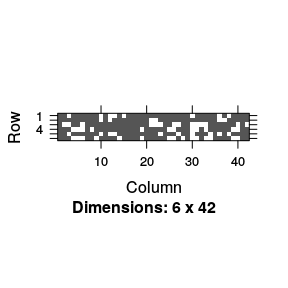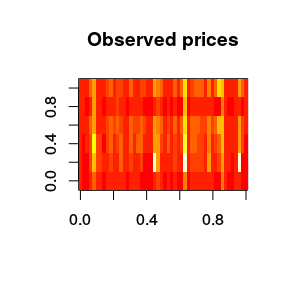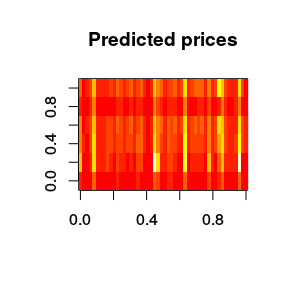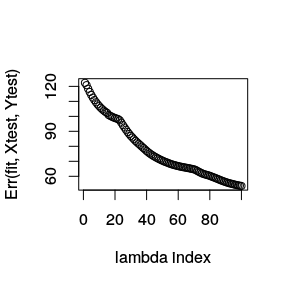# Quick Start

## Quick Start (for lsgl version 1.3.6)

### 1. Load the msgl library in R

library(lsgl)

Load data containing N samples and p features (covariates) and a response matrix containing K responses for each sample:

X <- # load design matrix (of size N x p)
Y <- # load response matrix (of size N x K)

For the purpose of this tutorial we will load a data set consisting of airline ticket prices

data(AirlineTicketPrices)
dim(X)
##  337 411
dim(Y)
##  337   6

Hence, p = 411, N = 337 and the dimension of the response K = 6, this implies that the model has 6*(411+1) = 2472 parameters.

Let us take out a small test set:

idx <- sample(1:nrow(X), size = 50)

Xtest <- X[idx, ]
Ytest <- Y[idx, ]
X <- X[-idx, ]
Y <- Y[-idx, ]

### 3. Estimate error using cross validation

Choose lambda (fraction of lambda.max) and alpha, with alpha = 1 for lasso, alpha = 0 for group lasso and alpha in the range (0,1) for spares group lasso.

Use lsgl::cv to estimate the error for each lambda in a sequence decreasing from the data derived lambda max to lambda * lambda max. Lambda max is the lambda at which the first penalized parameter becomes non-zero. A smaller lambda will take longer to fit and include more features. The following command will run a 10 fold cross validation for each lambda value in the lambda sequence using 2 parallel units (using the foreach and doParallel packages.

cl <- makeCluster(2)
registerDoParallel(cl)

# Do cross validation -- this may take some time
fit.cv <- lsgl::cv(X, Y, fold = 10, alpha = 0.5, lambda = 0.01, use_parallel = TRUE)
##
## Running lsgl 10 fold cross validation
##
##  Samples:  Features:  Models:  Groups:  Parameters:
##        287        412        6      412       2.472k
stopCluster(cl)

(for the current version no progress bar will be shown)

Get a summery of the validated models. We have now cross validated the models corresponding to the lambda values, one model for each lambda value. We may get a summery of this validation by doing:

fit.cv
##
## Call:
## lsgl::cv(x = X, y = Y, alpha = 0.5, lambda = 0.01, fold = 10,
##     use_parallel = TRUE)
##
## Models:
##
##  Index:  Lambda:  Features:  Parameters:  Error:
##        1    1.000          3           18     135
##       20    0.413        4.6         27.6     105
##       40    0.163        9.8         56.7      80
##       60    0.064       14.9         85.6      67
##       80    0.025       28.2        138.5      59
##      100    0.010       44.5        193.2      53
##
## Best model:
##
##  Index:  Lambda:  Features:  Parameters:  Error:
##      100     0.01       44.5        193.2      53

Hence, the best model is obtained using lambda index 100 and it has a cross validation error of 52.63. The expected number of selected features is 44.5 and the expected number of parameters is 193.2.

### 4. Fit the final model

Use lsgl to fit a final model.

fit <- lsgl::fit(X, Y, alpha = 0.5, lambda = 0.01)
##
## Running lsgl
##
##  Samples:  Features:  Models:  Groups:  Parameters:
##        287        412        6      412       2.472k

Get a summery of the estimated models

fit
##
## Call:
## lsgl::fit(x = X, y = Y, alpha = 0.5, lambda = 0.01)
##
## Models:
##
##  Index:  Lambda:  Features:  Parameters:
##        1    1.000          3           18
##       20    0.413          6           36
##       40    0.163         10           58
##       60    0.064         16           93
##       80    0.025         26          126
##      100    0.010         42          187

Take a look at the estimated models. As we saw in the previous step the model with index 100 had the best cross validation error, we may take a look at the included features using the command:

features(fit)[[best_model(fit.cv)]][1:10] # Ten first non-zero features in best model
##   ""                  "'ALLnumquotesA'"   "'ALLnumquotesA'l1"
##   "'ALLnumquotesA'l7" "'ALLminp0'"        "'ALLminp0'l1"
##   "'ALLnumquotes4'l2" "'aAAminp0'l1"      "'aAAminp0'l2"
##  "'aCOminpA'"

Hence 42 features are included in the model, this is close to the expected number based on the cross validation estimate.

The sparsity structure of the parameters belonging to these 42 features may be viewed using

image(parameters(fit)[[best_model(fit.cv)]])We may also take a look at the estimate parameters (or coefficients)

coef(fit, best_model(fit.cv))[,1:5] # First 5 non-zero parameters of best model
## 6 x 5 sparse Matrix of class "dgCMatrix"
##                                'ALLnumquotesA' 'ALLnumquotesA'l1
## LBL+ALLminpA+fut_001  91.82393   -1.236003e-05       .
## LBL+ALLminp0+fut_001 -53.14964   -5.374433e-06       0.006200295
## LBL+aCOminpA+fut_001 -24.04310    9.595046e-06       0.019909180
## LBL+aFLminpA+fut_001  54.48162   -1.332136e-05       .
## LBL+aUAminpA+fut_001 -49.44576    8.619017e-06       0.024576489
##                      'ALLnumquotesA'l7 'ALLminp0'
## LBL+ALLminpA+fut_001      -0.008327093 0.05548541
## LBL+ALLminp0+fut_001      -0.023200631 0.28014445
## LBL+aCOminpA+fut_001       .           .
## LBL+aFLminpA+fut_001      -0.008033362 0.03438073
## LBL+aUAminpA+fut_001       .           .

If we count the total number of non-zero parameters in the model we get, in this case 187 which is close to the expected based on the cross validation estimate.

### 6. Use your model for predictions

Load test data containing M samples and p features.

Xtest <- # load matrix with test data (of size M x p)

Use the final model to predict the price vector of the M=50 samples in Xtest.

res <- predict(fit, Xtest)

Plot predicted and true response

image(Ytest, main = "Observed prices")image(res\$Yhat[[best_model(fit.cv)]], main = "Predicted prices")Compute the error rates on the test set

plot(Err(fit, Xtest, Ytest), xlab = "lambda index")